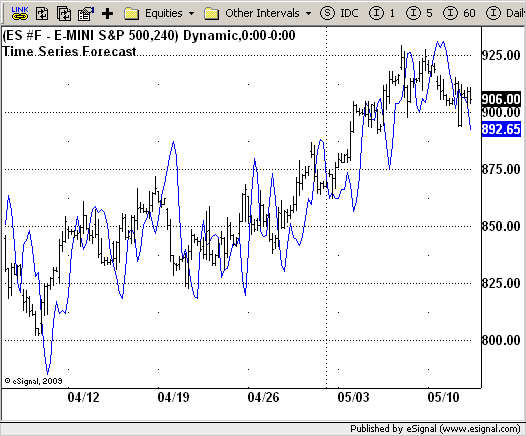# Time Series Forecast (TSF)

ICE Data Services -

TimeSeriesForecast.efs
EFSLibrary - Discussion Board

File Name: TimeSeriesForecast.efs

Description:
Time Series Forecast (TSF)

Formula Parameters:

• nLength : 5
• nBarPlus : 7

Notes:
This technical indicator is based on linear regression analysis.
The value of TSF for each bar is based on a regression analysis of the preceding N bars. N is called the regression period in the setup window for TSF. The user specifies a forecast period F. F is used to derive a predicted (forecasted) price value F periods in the future based on the slope of the regression line for the preceding N periods.

TimeSeriesForecast.efsEFS Code:

```/*********************************
Provided By:
eSignal (Copyright c eSignal), a division of Interactive Data
Formula Script (EFS) is for educational purposes only and may be
modified and saved under a new file name.  eSignal is not responsible
for the functionality once modified.  eSignal reserves the right
to modify and overwrite this EFS file with each new release.

Description:
Time Series Forecast (TSF)

Version:            1.0  05/12/2009

Formula Parameters:                     Default:
nLength                             5
nBarPlus                            7

Notes:
This technical indicator is based on linear regression analysis.
The value of TSF for each bar is based on a regression analysis
of the preceding N bars. N is called the regression period in
the setup window for TSF. The user specifies a forecast period
F. F is used to derive a predicted (forecasted) price value F
periods in the future based on the slope of the regression line
for the preceding N periods.

**********************************/
var fpArray = new Array();
var bInit = false;

function preMain() {
setPriceStudy(true);
setStudyTitle("Time Series Forecast");
setCursorLabelName("TSF");
var x = 0;
fpArray[x] = new FunctionParameter("nLength", FunctionParameter.NUMBER);
with(fpArray[x++]) {
setLowerLimit(1);
setDefault(5);
}
fpArray[x] = new FunctionParameter("nBarPlus", FunctionParameter.NUMBER);
with(fpArray[x++]) {
setLowerLimit(1);
setDefault(7);
}
}

var xTimeSeriesForecast = null;

function main(nLength, nBarPlus) {
var nBarState = getBarState();
var nTimeSeriesForecast = 0;
if (nBarState == BARSTATE_ALLBARS) {
if (nLength == null) nLength = nLength;
if (nBarPlus == null) nLength = nBarPlus;
}
if (bInit == false) {
xTimeSeriesForecast = efsInternal("Calc_TSF", nLength, nBarPlus);
bInit = true;
}
nTimeSeriesForecast = xTimeSeriesForecast.getValue(0);
if (nTimeSeriesForecast == null) return;
return nTimeSeriesForecast;
}

var xClose = null;
var bSecondInit = false;

function Calc_TSF(nLength, nBarPlus) {
var SL = 0;
var TSF = 0;
var SumBars = nLength * (nLength - 1) * 0.5;
var SumSqrBars = (nLength - 1) * nLength * (2 * nLength - 1) / 6;
var Sum1 = 0;
var SumY = 0;
var i = 0;
var Slope = 0;
var Intercept = 0;
if (getCurrentBarCount() <= nLength) return;
if(bSecondInit == false){
xClose = close();
bSecondInit = true;
}
for (i = 0; i < nLength; i++) {
Sum1 += i * xClose.getValue(-i);
SumY += xClose.getValue(-i);
}
var Sum2 = SumBars * SumY;
var Num1 = nLength * Sum1 - Sum2;
var Num2 = SumBars * SumBars - nLength * SumSqrBars;
if (Num2 == 0) return;
Slope = Num1 / Num2;
SL = Num1 / Num2;
Intercept = (SumY - Slope * SumBars) / nLength;
TSF = Intercept + Slope * (nLength - 1 - nBarPlus);
return TSF;
}```